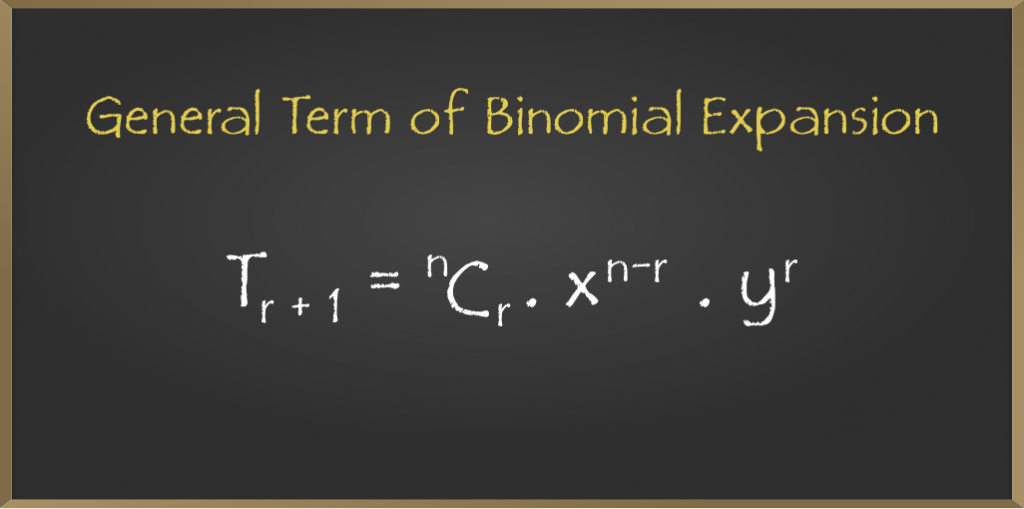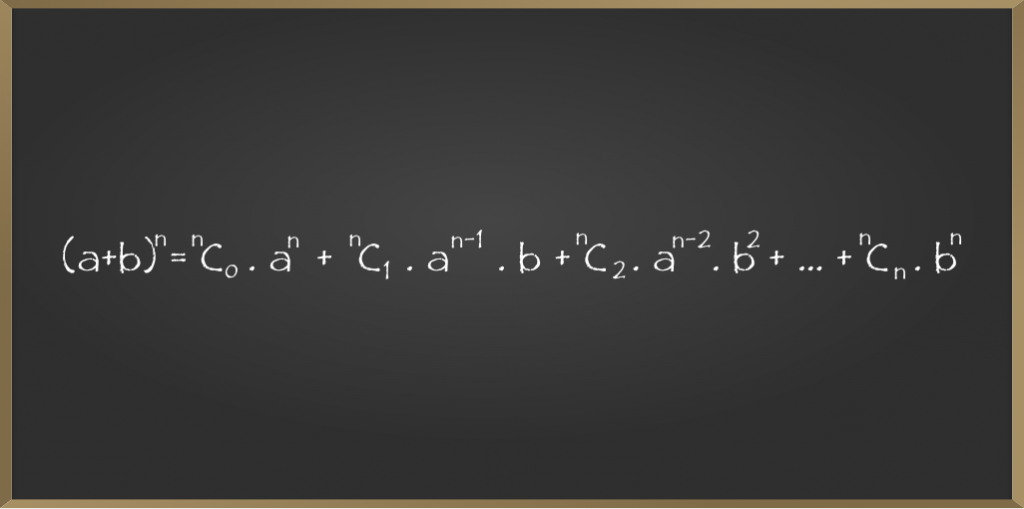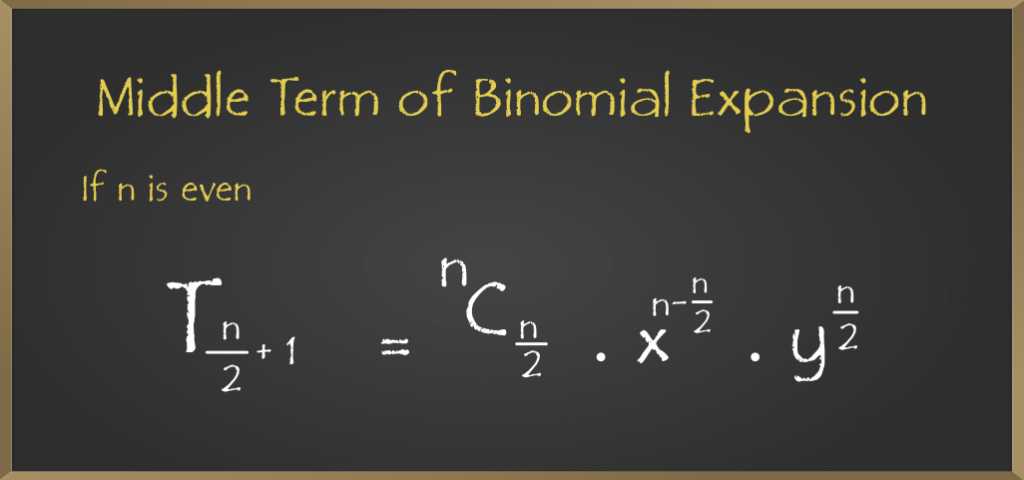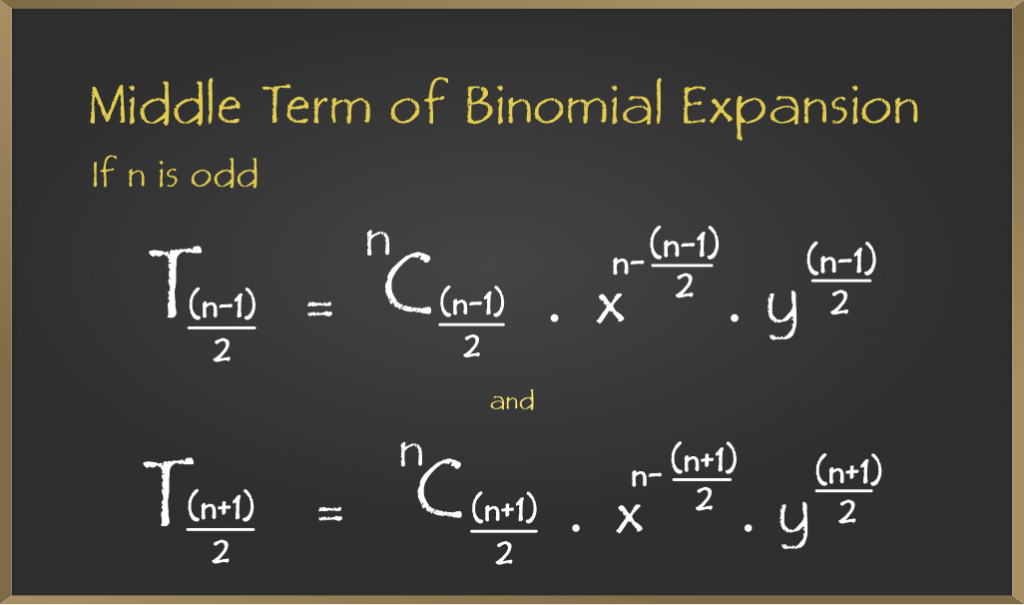# General and Middle Terms – Binomial Theorem – Class 11 Maths

• Last Updated : 27 Oct, 2020

Binomial theorem or expansion describes the algebraic expansion of powers of a binomial. According to this theorem, it is possible to expand the polynomial “(a + b)n into a sum involving terms of the form “axzyc, the exponents z and c are non-negative integers where z + c = n, and the coefficient of each term is a positive integer depending on the values of n and b.

Example: If n = 4

Attention reader! All those who say programming isn't for kids, just haven't met the right mentors yet. Join the  Demo Class for First Step to Coding Coursespecifically designed for students of class 8 to 12.

The students will get to learn more about the world of programming in these free classes which will definitely help them in making a wise career choice in the future.

(a + b)4 = a4 + 4a3y + 6a2b2 + 4ab3 + b

## General Term of Binomial Expansion

The General Term of Binomial Expansion of (x + y)n is as follows• Tr+1 is the General Term in the binomial expansion
• The General term expansion is used to find the terms mentioned in the above formula.
• To find the terms in the binomial expansion we need to expand the given expansion.
• Suppose (a + b)n is the equation then the series of its binomial expansion will be as follows:• The first term of the series is T1 = nC0.an
• The second term of the series is T2 = nC1.an-1.b
• The third term of the series is T3 = nC2.an-2.b2
• The nth term of the series is Tn= nCn.bn

### Sample Problems on General Term

Example 1: Find (r+1)th  term for the given binomial expansion (x + 2y)5

Solution:

Given expansion is (x + 2y)5

a = x, b= 2y, n = 5

The formula for (r+1)th is  nCr .an – r.br

(r+1)th term =5Cr.x5 -r.2yr

Example 2: Find (r+1)th term for the given binomial expansion (a + 2b)7

Solution:

Given expansion is (a + 2b)7

a = a, b = 2b, n = 7

The formula for (r+1)th is  nCr .an – r.br

(r+1)th term = 7Cr.a7 -r.br

Example 3: Find (r + 1)th term for the given binomial expansion (6p + 2q)12

Solution:

Given expansion is  (6p + 2q)

a = 6p, b = 2q, n = 12

The formula for (r+1)th is  nCr .an – r.br

(r + 1)th term = 12Cr.6p12 -r.2qr

## Middle Term of the Binomial Expansion

If (x + y)n =  nCr.xn – r.yr  , it has (n + 1) terms and the middle term will depend upon the value of n.

We have two cases for the Middle Term of a Binomial Expansion:

### If n is Even

If n is the even number then we make it into an odd number and consider (n + 1) as odd and (n/2 + 1) is the middle term. In simple, if n is even then we consider it as odd.

Suppose n is the even so, (n + 1) is odd. To find out the middle term :

Consider the general term of binomial expansion i.e.• Now we replace “r ” with “n/2” in the above equation to find the middle term
• Tr+1 = Tn/2 + 1
• Tn/2 + 1 = nCn/2.xn – n/2.yn/2

### Sample Problems on Middle Terms

Example 1: Find the middle of the following binomial expansion (x + a)8

Solution:

Given expansion is (x + a)8

n = 8, we consider the expansion has (n + 1) terms so the above expansion has (8 + 1) i.e 9 terms

we have T1, T2, T3, T4, T5, T6 , T7, T8, T9.

Tr+1 = Tn/2 + 1 = nCn/2.xn – n/2.Yn/2

T8/2 + 1 = 8C8/2.x8-8/2.a8/2

T5 = 8C4.x4.a4 is the required middle term of the given binomial expansion.

Example 2: Find the middle of the following binomial expansion (x + 3y)6

Solution:

Given expansion is (x + 3y)6

n = 6, we consider the expansion has (n + 1) terms so the above expansion has (6 + 1) i.e 7 terms

we have T1, T2, T3, T4, T5, T6 , T7.

Tr+1 = Tn/2 + 1 = nCn/2.xn – n/2.Yn/2

T6/2 + 1 = 6C6/2.x6-6/2.3y6/2

T4 = 6C3.x3.3y3 is the required middle term of the given binomial expansion.

Example 3: Find the middle of the following binomial expansion (2x + 5y)4

Solution:

Given expansion is  (2x – 5y)4

n = 4, we consider the expansion has (n + 1) terms so the above expansion has (4 + 1) i.e 5 terms

we have T1, T2, T3, T4, T5.

Tr+1 = Tn/2 + 1 = nCn/2.xn – n/2.Yn/2

T4/2 + 1 = 4C4/2.2x4-4/2.5y4/2

T3 = 4C2.x2.5y2 is the required middle term of the given binomial expansion.

### If n is Odd

If n is the odd number then we make it into an even number and consider (n + 1) as even and (n + 1/2), (n + 3/2) is the middle terms. In simple, if n is odd then we consider it as even.

We have two middle terms if n is odd. To find the middle term:

Consider the general term of binomial expansion i.e• In this case, we replace “r” with the two different values
• One term is (n + 1/2) compare with (r + 1) terms we get

r + 1 = n + 1/2

r = n + 1/2 -1

r = n -1/2

• Second middle term , compare (r + 1) with (n + 3/2) we get

r +1 = n +3/2

r = n + 3/2 – 1

r = n + 1/2

The two middle terms when n is odd are (n – 1/2) and (n + 1/2).

### Sample Problems on Middle Terms

Example 1: Find the middle terms of the following binomial expansion (x + a)9

Solution:

Given expansion is (x + a)9

a = x, b = a, and n = 9

Middle terms will be (n – 1)/2 and (n + 1)/2

Tr + 1 =T n – 1/2 and Tn + 1/2

First middle term:

Tr + 1 = Tn – 1/2 = 9C(n – 1)/2.x9 – (n – 1)/2.a(n – 1)/2

T(9 – 1/2) = 9C(9 – 1)/2.x9 – (n – 1)/2.a(n – 1)/2

T4 = 9C4.x5.a4

Second middle term:

Tr + 1 = Tn + 1/2 =  9C(n + 1)/2.x9 – (n + 1)/2.a(n + 1)/2

T(9 + 1)/2 = 9C(9 + 1)/2.x9 – (9 + 1)/2.a(9 + 1)/2

T5 = 9C5.x4.a5

The middle terms of expansion are T4, and T5.

Example 2: Find the middle terms of the following binomial expansion (4a + 9b)7

Solution:

Given expansion is (4a + 9b)7

a = 4a,b = 9b, and n = 7

middle terms will be (n -1/2) and (n + 1/2)

Tr + 1 =T n – 1/2 and Tn + 1/2

First middle term:

Tr + 1 = Tn – 1/2 = 7C(n – 1)/2.4a7 – (n – 1)/2.9b(n – 1)/2

T(7 – 1/2) = 7C(7 – 1)/2.4a7 – (7 – 1)/2.9b(7 – 1)/2

T3 = 7C3.4a4.9b3

Second middle term:

Tr + 1 = T(n + 1)/2 =  7C(n + 1)/2.4a7 – (n + 1)/2.9b(n + 1)/2

T(7 + 1)/2 =  7C(7 + 1)/2.4a7 – (7 + 1)/2.9b(7 + 1)/2

T4 = 7C4.4a3.9b4

The middle terms of expansion are T3, and T4.

Example 3: Find the middle terms of the following binomial expansion (2x + 8y)5

Solution:

Given expansion is (2x + 8y)5

a = 2x,b = 8y, and n = 5

Middle terms will be (n – 1)/2 and (n + 1)/2

Tr + 1 = T (n – 1)/2 and T(n + 1)/2

First middle term:

Tr + 1 = T(n – 1)/2 = 5C(n – 1)/2.2x5 – (n – 1)/2.8y(n – 1)/2

T(5 – 1)/2 = 5C(5 – 1)/2.2x5 – (5 – 1)/2.8y(5 – 1)/2

T2 = 5C2.2x3.8y2

Second middle term:

Tr + 1 = T(n + 1)/2 =  5C(n + 1)/2.2x5 – (n + 1)/2.8y(n + 1)/2

T(5 + 1)/2 =  5C(5 + 1)/2.2x5 – (5 + 1)/2.8y(5 + 1)/2

T3 = 5C3.2x2.8y3

The middle terms of expansion are T2, and T3.

My Personal Notes arrow_drop_up